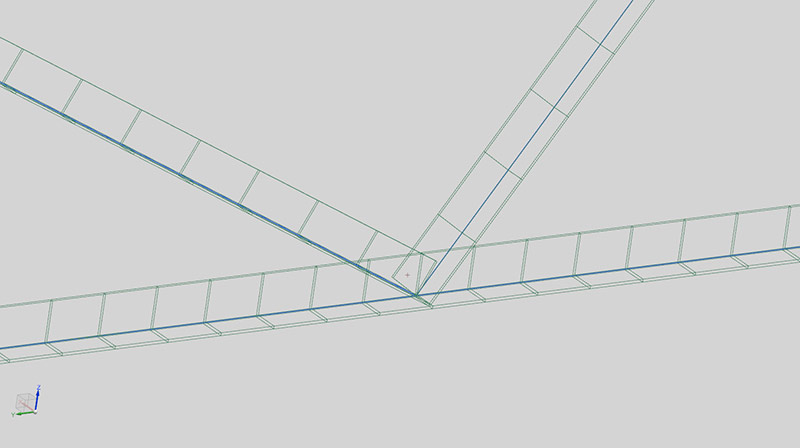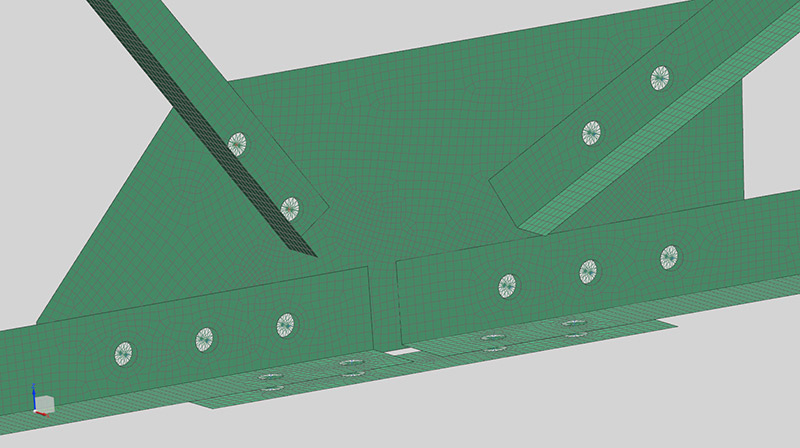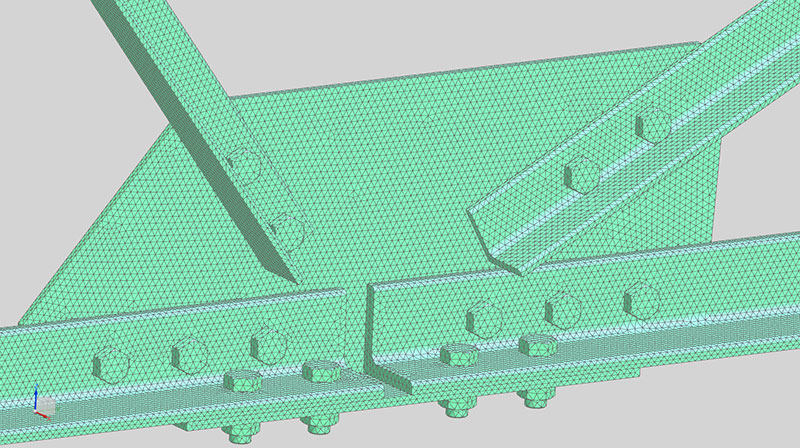WRITTEN BY:

More precisely: “How accurately can an FEA (Finite Element Analysis) predict the real response of a structure?” Let’s start by introducing the typical process (chain) of getting from a real structure to an FEA model with results:

1. Set up 3D CAD (Computer Aided Design) geometry of the real structure

2. Simplify the CAD by removing unnecessary details

3. Add connections between parts (bolts, welds etc.)

4. Create a discretized representation of the simplified CAD model

5. Specify material models to be used and material constants for these

6. Apply constraints that attempts to match the real structure’s attachments

8. Choose which physics to include and which to exclude

9. Solve the model

10. Interpret the results

A perfect match between the real structure and the FEA model will only be possible if none of these introduce any error. In other words, if the 3D CAD perfectly matches the real geometry, no simplifications are made, the discretization error is negligible, materials are perfect etc.

This is obviously impossible, so let’s look at each point and attempt to quantify what is possible.

When creating a CAD model of an existing part, it is possible to obtain a model that is extremely accurate. We tend to do things the other way around though: We design in CAD then manufacture the part.

We have a number of avenues for discrepancies to creep in between CAD and the real structure which will be manufactured:

• Standard sections have tolerances. As an example, for 5mm plate a tolerance of ±0.35mm (±7%) is allowed

• In CAD models, welding is seldom detailed in 3D

• All machining operations, from milling to drilling have tolerances.

• Assembly can cause discrepancies (weld sized, bolt preloads etc.)

The better controlled the machining and assembly of a structure, the more accurately the CAD can represent the real structure. Below are some examples:

• Machining a component using a highly accurate Computer Numerically Controlled (CNC) mill in a thermally controlled environment may result in a real part that is within a couple of μm of the CAD model. For all intents-and-purposes, the CAD error would be negligible.

• If standard sections and plates are used, a significant variation in properties becomes possible, even likely. This impacts FEA to Real-Structure accuracy, but also causes scatter in the response of real structures: Ten structures would yield ten different answers.

• A single component is much easier to model accurately. Each additional part introduces a little bit of scatter, decreasing CAD (and FEA) fidelity.

In FEA, we tend to simplify the CAD to some extent. The intent here is to remove features in the CAD which will cause excessive model setup- and run-time, but which will have minimal impact on the results.

It is easy to remove the wrong ones though: If a small hole is removed from a large plate, the overall deflection will not change significantly. However, the stress near the hole will change dramatically. If this is in an area with a low average stress, this may not be important. If this area is already highly stressed, removing it will remove an area with a potentially very high stress level.

The error introduced due to CAD simplification is therefore up to the Analyst. Engineering judgment will have to be exercised in order to estimate error.

## Connection modelling

For assemblies, the connection between the parts of the assembly is critical to how the real structure behaves. Accurate modelling of the connections is required if an accurate FEA answer is required.

1-D Mesh2-D Mesh3-D MeshFigure 1 CAD model and various FEM representations

## FEM discretization

The FEM discretization is often referred to as the FEA Mesh. The error here can be attributed to at least two distinct sources:

### The element type

A solid element has the potential to be the most accurate. This is because it has the ability to calculate a complete 3D stress state at each node in the model.

In a typical plate-structure, the stress through the thickness will be small enough compared to the other directions that this component can be neglected. The shell element was designed to cater for this in a very efficient manner.

For slender structures, a beam formulation can be used as the stress and displacement is dominated by axial, shear and bending behavior of the structure. The beam element is again ideal to represent the behavior of the structure.

Using a shell element in a situation where shell-theory is violated will result in wrong answers. Using a solid element for a plate-structure or a beam-structure will result in a more accurate answer than using a shell element or beam element respectively as long as the mesh is converged in all cases. This is because it will capture the stress components which cannot be represented by a shell / beam element. They will however be very expensive in runtime if these components were not important and of interest as the number of elements will be significantly higher.

### Mesh convergence

Mesh convergence refers to how well the discretization in use represents reality. This is determined by combination of items:

• Element shape

• Tetrahedron (Tet), Triangular prism (Penta), Pyramid or hexahedron (Hex) for solid elements

• Element order

• Usually first or second order elements are used

• For P-Elements, the order of each edge / face can vary independently

• Element formulation and integration order

• Full-integration vs. reduced integration

• Iso-parametric vs. Assumed strain formulation

• Element distortion

• Mesh density (i.e. how fine is the mesh)

The combination of element type, shape, order, formulation, distortion and mesh density determines the mesh convergence.

The FEM discretization error is therefore entirely up to the Analyst’s choice of element shape, class, order and mesh-refinement. If care is taken, this can be reduced to extremely low levels. The opposite is true as well – it is very easy to under-predict displacement by more than one order of magnitude and stress by several orders of magnitude by using a coarse first-order tri-element mesh.

## Material properties

The choice of material model and the accuracy of the material data have a significant impact on FEA accuracy. As an example, the elastic modulus of steel varies from somewhere around 187 GPa to about 207 GPa. While there are steels outside this range, most commonly used steel falls somewhere in this range.

Using the wrong elastic modulus for a material has an interesting impact on the results. If we consider a linear structure which contains only a single material of elastic modulus EPhysical / EFEA in the FEA model, then:

• Applying a known load to the structure will yield the correct stress, but the predicted deflection will be EPhysical / EFEA times the actual response

• Applying an enforced motion to the structure will yield the correct deformation, but the predicted stress will be EFEA / EPhysical times the actual stress

In other words, using the wrong elastic-modulus can result in some predicted responses having no error while other predicted responses will be out by the fraction of error in the material properties used.

## Constraints

Read this carefully: Since a constraint is infinitely rigid, they add an infinite amount of stiffness to your structure.

In other words, if constraints are not used with utmost care, they can cause infinitely wrong answers. Instead of producing a scaled result like an error in load magnitude or material constant, a wrong constraint is more likely to cause a result that doesn’t match actual response in either distribution or magnitude.

Applying correct constraints are therefore very important for correct (or even reasonable) results.

It should be intuitively obvious that scaling the applied load will scale the results by the same ratio if the calculation is linear.

In the same way, applying the wrong load distribution would also result in results that are wrong. If the load distribution used and the actual are statically equivalent, the results away from the loaded area should at least be correct though. See figure 2

## Physics modelled

By physics, we’re referring to whether the analysis takes into consideration the behavior of everything that plays a role in the response of the real system. Some examples of questions to be asked about the response of the system are the following:

• Is the actual response linear or nonlinear:

• Are large rotations present in the response?

• Does regions come into contact or separate?

• Is any material behavior nonlinear (Plasticity, creep, failure etc.)?

• Do the load magnitude and/or direction change due to the deformation?

• Are thermal effects significant?

• Is the response static or dynamic?

If any of these are significant, but not included in the analysis, the calculated response will per definition not take the neglected physics into account.

## Solving the model

The FEA analysis code (solver) to some extent plays a role in all of the previous due to the specific element types it supports, material models, load types etc. These are considered already, so are not too important from the FEA solver’s perspective. Whether the solver has the material models or physics needed is a capability question instead of an accuracy question.

With the exception of bugs in the solver (and solver limitations), all FEA codes should give very close to the same answer if all the previous sources of error are eliminated. All commercial FEA codes spend a lot of time and effort to find and trace bugs, so the probability of a user triggering one is small. As I’m not too familiar with open-source codes, I can’t comment: I expect that they put in similar levels of effort to trace and fix bugs.

In other words, the solver can cause bad answers, but is very unlikely to do so – at least for the simpler models. The previously mentioned sources of error tend to be orders of magnitude greater than solver error. The solver error can probably be ignored until extreme effort has been expended to model a real system “perfectly”.

## Results interpretation

Strange as it sounds, it is possible for multiple engineers to look at the exact same results and interpret it differently. If we assume the results perfectly match the response of the real system, the only difference in predicted behavior is in whether the structure will “fail”. Fail here can be anything from material that has yielded and should not have, fatigue fracture or static fracture.

To resolve these, a better understanding of material response is required, not a better understanding of FEA.

## Conclusion

FEA can yield spectacularly accurate results. Less than 1% error is entirely possible. Is this probable? Definitely not!

In order to have a highly accurate finite element analysis, extreme attention to detail is required. The FEA model has to be near perfect in terms of ALL of the following:

• The finite element model should be an accurate geometric representation of the real structure, with element types that can represent the behavior correctly and have a converged mesh

• The material model and properties have to accurately match the real material’s characteristics